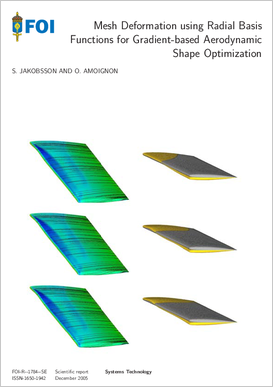# Mesh deformation using radial basis functions for gradient-based aerodynamic shape optimizationAuthors:

Publish date: 2005-01-01

Report number: FOI-R--1784--SE

Pages: 36

Written in: English

## Abstract

Gradient-based aerodynamic shape optimization using Computational Fluid Dynamics (CFD), and time dependent problems in aeroelasticity, that is, coupled calculations between Computational Structural Mechanics (CSM) and CFD, require repeated deformations of the CFD mesh. An interpolation scheme, based on Radial Basis Functions (RBF), is devised in order to propagate the deformations from the boundaries to the interior of the CFD mesh. This method can lower the computational costs due to the deformation of the mesh, in comparison with the usual Laplace smoothing. Moreover, the algorithm is independent of the mesh connectivities. Therefore, structured and unstructured meshes are equally treated as well as hybrid meshes. The application of this interpolation scheme in problems of aerodynamic shape optimization is also carefully investigated. When the optimization is executed by a gradient-based algorithm the cost function is differentiated with respect to the design parameters in order to obtain the gradient. The gradient is most efficiently and accurately calculated by solving a certain adjoint equation derived from the discretized flow equations. The calculation of the gradient, which is detailed in this presentation, involves the Jacobian matrix of the mesh deformation. Finally, we present the results of an optimization of the ONERA M6 wing at transonic speed using the interpolation algorithm. The results are used for comparison with another technique of mesh deformation. The quality of the mesh obtained by the new algorithm, and the interpolation error, are analyzed with respect to the parameters of the interpolation scheme: the type of RBF, the RBFs shape parameter, and the sets of control points.

Share page on social media